## Friday, December 30, 2005

### To shoehorn an infinite sum of functions into the exponential function

Expressing the exponential function as an infinite sum of algebraic functions: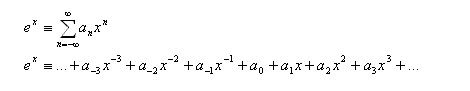We will attempt to shoehorn the exponential function, elegantly expressed as e^x, into the infinite sum by finding suitable values for all a’s. Or more accurately, try to shoehorn the infinite sum of functions to fit into a simple function.

One of the better known characteristics of the exponential function is that its derivative is the function itself. It is the only function that exhibits this remarkable property, and we will take advantage of this.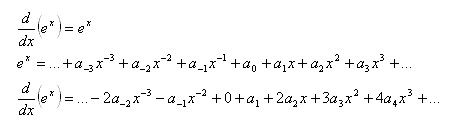As already mentioned, the derivative of the exponential function is itself, thus the above two expressions are equal.

In the process of differentiating, something happens around the a(0) term: the constant function becomes zero after differentiating, and there is nothing left to pair with a(-1)x^-1.

Comparing the coefficients of all powers of x (all terms with x^2 must be the same, and all terms with x^3 must be the same, and so on for all x^n):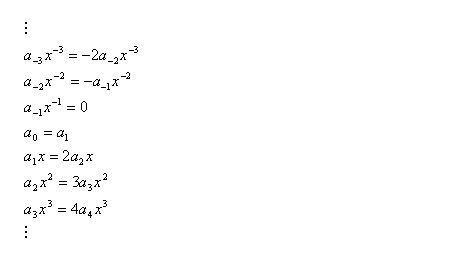The left side of the equations come from the original function, and the right side from the derivative.

The zero at a(-1) appears to cause all a’s with negative subscripts to be zero. This will be proven by induction- firstly, show that a(-1) is zero. Then, show that a(n-1) is zero when a(n) is zero, for all negative n integers.

a(-1) is zero, which is already shown.
Then, establish the relationship between the coefficients of powers of x. This is merely differentiation of a power function.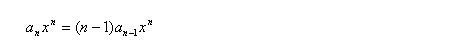Since x is not necessarily zero, and (n-1) is never zero for negative values of n, if a(n) is zero, then a(n-1) will be zero.

Putting these two parts together, all a’s with negative subscripts can be proven to be zero, since a(-1) is zero, then a(-2) is zero, then a(-3) is zero…

Now, the infinite sum can be simplified slightly by removing all a’s with negative subscripts.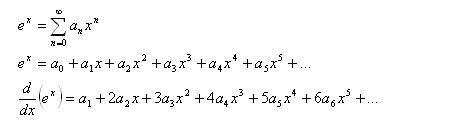Again, comparing the coefficients of the powers of x: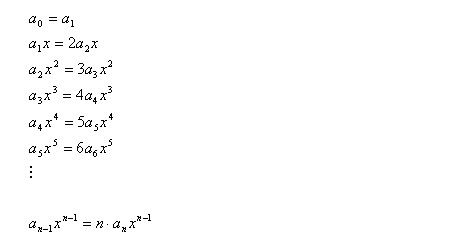Looking at the set of equations, one can see that all the values of the a’s are closely connected. If a(0) is known, then a(1) is known, then a(2) is known, then a(3) is known…

The task now is to extract the value of any one of the a’s, and the value of all others will fall into place. The simplest would be a(0).

The exponential function’s function value is 1 when it’s argument is 0.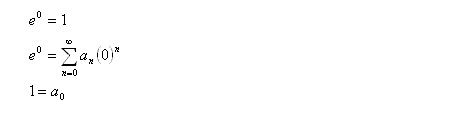All the x’s and their coefficients vanish into thin air when x is given the value of zero, leaving a(0) standing very alone to accept it’s fate as one.

Using proof by induction, it can be easily shown that a(n) = 1/n! for all non-negative values of n (bearing in mind that 0! = 1).

First, show that a(0) is 1, which has already been done.

Then show that if a(n-1) = 1/(n-1)!, then a(n) = 1/n!.

Using the relationship between the coefficients of the powers of x,Now, we are ready to reveal the transcendental function e^x as an infinite sum of algebraic functions, as an infinite-order polynomial.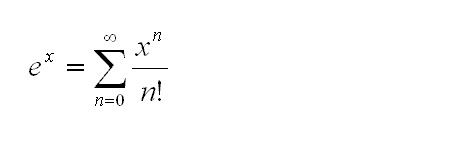end note:

I had previously found the value of e to be the infinite sum of the factorials inversed, using the Taylor series expansion. From that earlier result, it is not apparent at all that the exponential function is as easy as sticking in a power of x into the summation. However, now that this stronger result has been shown, that earlier result would not surprise anyone.

From a certain point of view, proof by induction is very much like a recursive function used in computer programming. Induction starts from the trivial case, and advances forward step by step; recursion starts from an arbitrary location as dictated by the function inputs, steps backwards until it arrives at the trivial solution, then marches back forward to the starting location to return the value.

Labels: